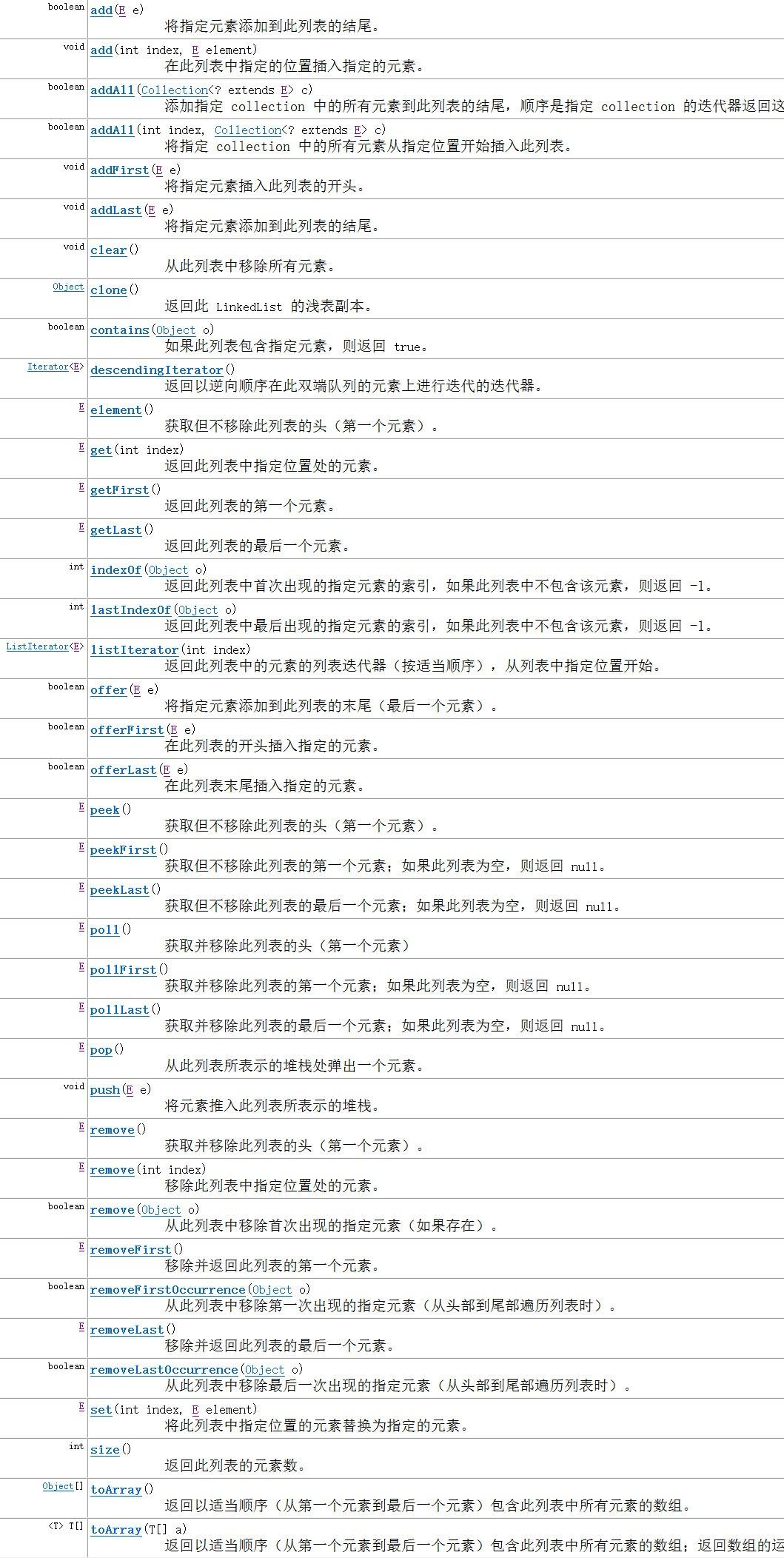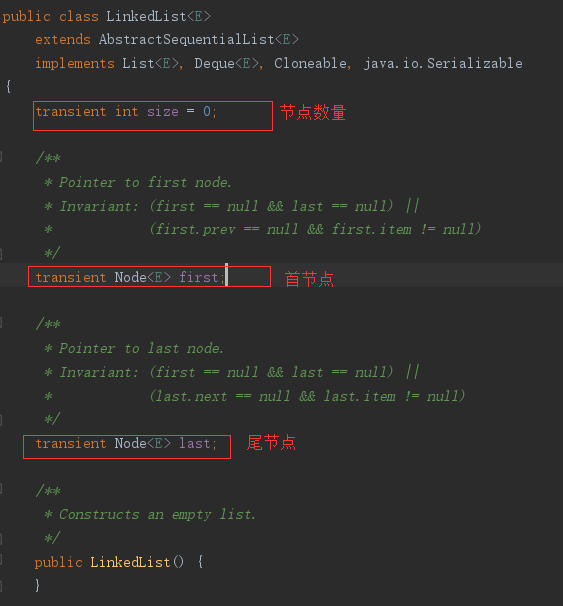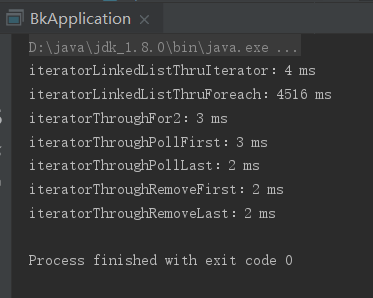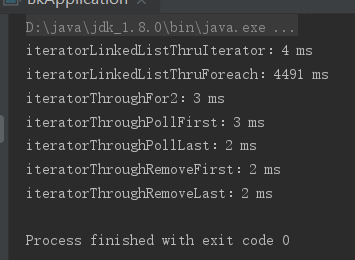1720 0 0## 2.双向链表的实现## 5.重要属性## 6.Node节点1.通过迭代器遍历。即通过Iterator去遍历

``````for(Iterator iter = list.iterator(); iter.hasNext();)
iter.next();``````

``````int size = list.size();
for (int i=0; i<size; i++) {
list.get(i);
}``````

``````for (Integer integ:list)
;``````

``````while(list.pollFirst() != null)
;``````

``````while(list.pollLast() != null)
;``````

``````try {
while(list.removeFirst() != null)
;
} catch (NoSuchElementException e) {
}``````

``````try {
while(list.removeLast() != null)
;
} catch (NoSuchElementException e) {
}``````

## 8.测试：

``````package com.dzqc.yx;

import java.util.Iterator;
import java.util.NoSuchElementException;

/*
*
* @author skywang
*/
public class BkApplication {
public static void main(String[] args) {

}

for (int i=0; i<100000; i++)

return llist;
}
/**
*/
if (list == null)
return ;

// 记录开始时间
long start = System.currentTimeMillis();

for(Iterator iter = list.iterator(); iter.hasNext();)
iter.next();

// 记录结束时间
long end = System.currentTimeMillis();
long interval = end - start;
}

/**
*/
if (list == null)
return ;

// 记录开始时间
long start = System.currentTimeMillis();

int size = list.size();
for (int i=0; i<size; i++) {
list.get(i);
}
// 记录结束时间
long end = System.currentTimeMillis();
long interval = end - start;
}

/**
*/
private static void iteratorThroughFor2(LinkedList<Integer> list) {
if (list == null)
return ;

// 记录开始时间
long start = System.currentTimeMillis();

for (Integer integ:list)
;

// 记录结束时间
long end = System.currentTimeMillis();
long interval = end - start;
System.out.println("iteratorThroughFor2：" + interval+" ms");
}

/**
*/
private static void iteratorThroughPollFirst(LinkedList<Integer> list) {
if (list == null)
return ;

// 记录开始时间
long start = System.currentTimeMillis();
while(list.pollFirst() != null)
;

// 记录结束时间
long end = System.currentTimeMillis();
long interval = end - start;
System.out.println("iteratorThroughPollFirst：" + interval+" ms");
}

/**
*/
private static void iteratorThroughPollLast(LinkedList<Integer> list) {
if (list == null)
return ;

// 记录开始时间
long start = System.currentTimeMillis();
while(list.pollLast() != null)
;

// 记录结束时间
long end = System.currentTimeMillis();
long interval = end - start;
System.out.println("iteratorThroughPollLast：" + interval+" ms");
}

/**
*/
private static void iteratorThroughRemoveFirst(LinkedList<Integer> list) {
if (list == null)
return ;

// 记录开始时间
long start = System.currentTimeMillis();
try {
while(list.removeFirst() != null)
;
} catch (NoSuchElementException e) {
}

// 记录结束时间
long end = System.currentTimeMillis();
long interval = end - start;
System.out.println("iteratorThroughRemoveFirst：" + interval+" ms");
}

/**
*/
private static void iteratorThroughRemoveLast(LinkedList<Integer> list) {
if (list == null)
return ;

// 记录开始时间
long start = System.currentTimeMillis();
try {
while(list.removeLast() != null)
;
} catch (NoSuchElementException e) {
}

// 记录结束时间
long end = System.currentTimeMillis();
long interval = end - start;
System.out.println("iteratorThroughRemoveLast：" + interval+" ms");
}

}``````java基础 913 javaHashtable一、构造方法1.publicHashtable()publicHashtable(){this(11,0.75f);}无参构造，初始化一个容量为11，负载因子为
java基础 819 一、HashSet底层实现从HashSet的源码中可以发现，它的底层维护了一个HashMap，在newHashSet的时候，构造方法中其实是new了一个HashMap。privatetransientHashMapE,Objectmap;publicHashSet(){map=newHashMap();}publicHashSet(Collection?extendsEc){map=newHash
java基础 2267 ArrayList的实现是一个动态数组，从源码中也可以看出。这就决定了他的查找效率高,可以直接通过下标访问。但是对于删除和增加元素，需要大量的移动元素，所以插入和删除的效率就很低。ArrayList不是线程安全的，只能用在单线程环境下，多线程环境下可以考虑用Collections.synchronizedList(Listl)函数返回一个线程安全的ArrayList类。构造方法：ArrayList
java基础 1255 javaTreeMap实现原理TreeMap的实现其实说简单也简单说复杂也复杂，说简单是因为TreeMap底层实现完全依靠红黑树这个数据结构，相比与HashMap来说TreeMap不用考虑